# x264 Codec Improvement

• Project head: Dr. Dmitriy Vatolin
• Implementation: Alexey Noskov, Alexander Parshin, Vladimir Popov

## Introduction

x264 is an open source codec of standard MPEG4 AVC/H.264, developed by programmers from all over the world. Nowadays, x264 is one of the best codecs of its standard, what is verified by different comparisons.

## Improvement description

We decided to improve ABR rate control mode. In this mode codec tries to compress a film with target average bitrate satisfying specified restrictions of maximum frame bitrate and buffer size.

Accumulated during previous frames’ compressions statistic is actively used by codec to improve effectiveness of adaptive models. But there is no statistical information on the first frames of the sequence. To solve this problem, codec uses some predefined values or values defined by user.

An analysis showed that these default values are more suitable to high bitrates. Therefore unfounded quality increase appears on low bitrates, that leads to deterioration of both quality of consequent frames and average quality of a movie.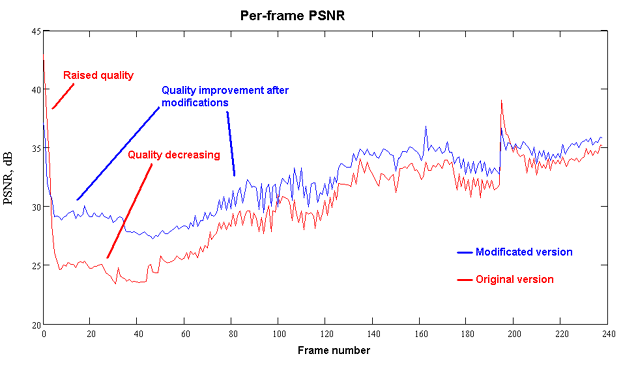Fig. 1. Per-frame values of PSNR metric before and after the modification. "Matrix" sequence.

To eliminate this drawback, we measured PSNR on different test sequences, and selected optimal values of these coefficients for different bitrates.

Two coefficients were modified in our measurements:

• start_qp - start quant coefficient. It is used to calculate quantifier for first frames, when codec accumulated no statistics. Unfortunately, codec can’t specify this coefficient for ABR mode from command line. So, we implemented this feature for testing.
• ipratio - quant coefficient modifier for I-frames. To calculate quantifiers for I-frames, codec uses results of accumulated statistics for P-frames, divided by this coefficient. This coefficient may be specified from a command line, as follows: ipratio = <number>

These parameters are used only before encoding start, so, our improvements are more significant for short sequences.

Following sequences were used for measurements:

• bbc - standard test sequence
• susi - standard test sequence
• battle - fragment from a movie “Terminator-2”
• matrix - fragment from a movie “Matrix”

Each sequence was compressed with bitrates 100, 225, 460, 938, 1340, 2340 kbps. For every bitrate coefficients were variated as follows: start_qp - from 24 to 48 with step 2, ipratio - from 0.50 to 1.50 with step 0.25. That allowed us to select optimal values for each bitarte and each sequence from the testing set. Range of parameters was selected to cover all possible optimal values with good accuracy and in acceptable time.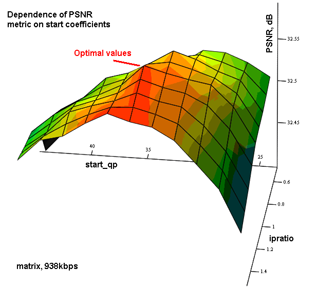Fig. 2. Dependence of average PSNR value on parameters
"start_qp" and "ipratio" for sequence "matrix" with 938 kbps bitrate.

As you can see on graph, quality of compressed movie mostly depends on start quant coefficient, and varying of I-frame modifier doesn’t cause a significant changes. So, it was decided to make changes only in start quantifier selecting.

Accumulated statistic was approximated by a simple function, dependent on specified bitrate, and calculated each time before compression starts, that leads to increase in quality and decrease in size of a movie.

To approximate experimental curve it was decided to use a power function of following type:

where a, b and c - unknown coefficients. Selection of coefficients was implemented using nonlinear Levenberg-Marquardt optimization. After optimization, function becomes:

## Results

After modifications codec’s work on low bitrates was improved. As a test, we used number of sequences, which didn’t participated in parameter selection. Most of them show that our modification outperform original version at low bitrates and has same average quality at middle and high bitrates. Sources of Febrary, 12, 2006 were used for this testing. We used following codecs parameters:

``````x264 --bitrate=<targer_bitrate> --fps=<fps> --me=umh --merange=32 --subme=6 --ref=16
--analyse=all --direct=spatial --pbratio=1.5 --bframes=3 --weightb -o <output_file> <input_file> <width>x<height>
``````

Following graphs show results at some sequences. “Matrix” is a sequence from our test set and “flower” sequence was not used for parameters selection.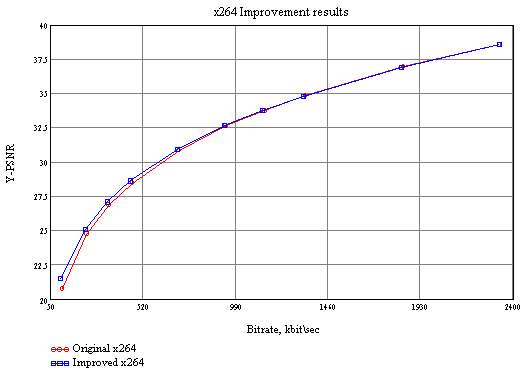Fig. 3 Results of codec x264 modification on "flower" sequence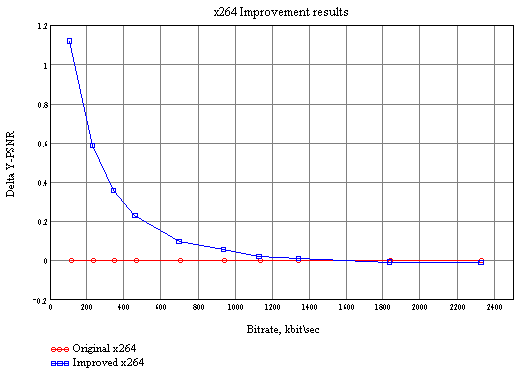Fig. 4 Results of codec x264 modification on "flower" sequence, Delta Y-PSNR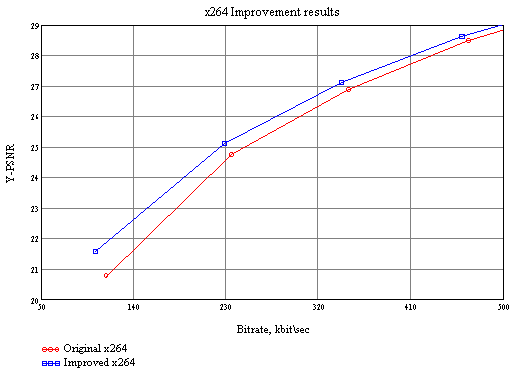Fig. 5 Results of codec x264 modification on "flower" sequence, low bitrates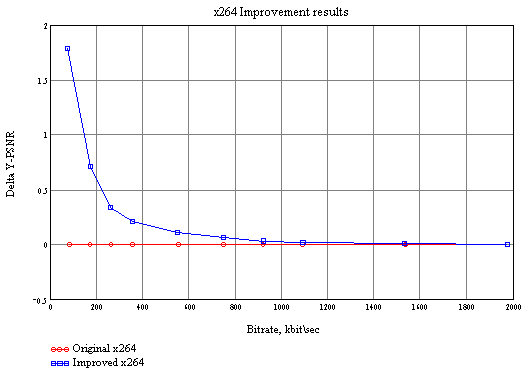Fig. 6 Results of codec x264 modification on "Matrix" sequence, Delta Y-PSNR

Codec comparisons with x264:

Codec’s web pages: